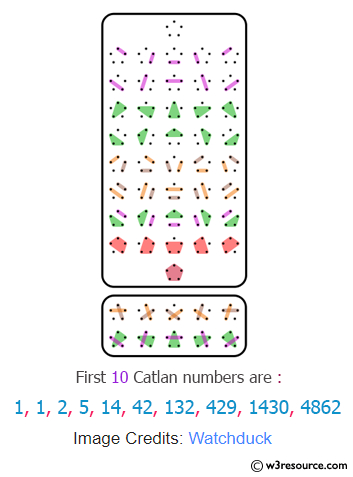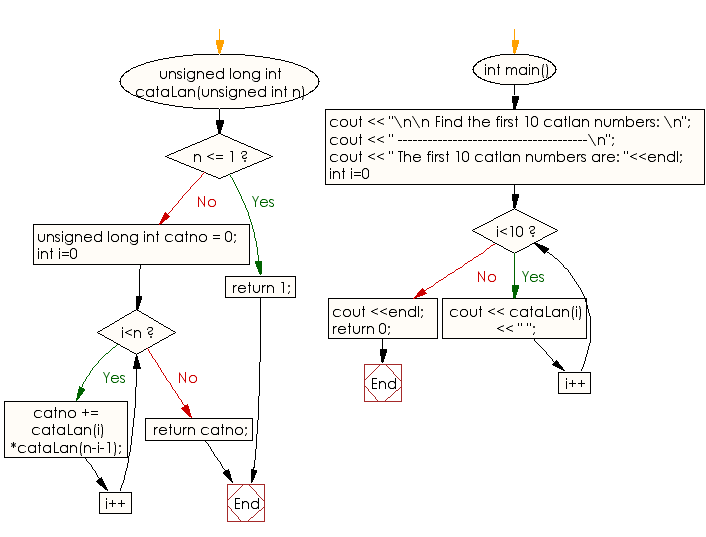﻿ C++ Exercises: Display the first 10 Catlan numbers - w3resource

# C++ Exercises: Display the first 10 Catlan numbers

## C++ Numbers: Exercise-15 with Solution

Write a program in C++ to display the first 10 Catlan numbers.

Pictorial Presentation:Sample Solution:

C++ Code :

``````#include<iostream>
using namespace std;
unsigned long int cataLan(unsigned int n)
{
if (n <= 1) return 1;
unsigned long int catno = 0;
for (int i=0; i<n; i++)
catno += cataLan(i)*cataLan(n-i-1);
return catno;
}
int main()
{
cout << "\n\n Find the first 10 Catlan numbers: \n";
cout << " --------------------------------------\n";
cout << " The first 10 Catlan numbers are: "<<endl;
for (int i=0; i<10; i++)
cout << cataLan(i) << " ";
cout <<endl;
return 0;
}
``````

Sample Output:

``` Find the first 10 Catlan numbers:
--------------------------------------
The first 10 Catlan numbers are:
1 1 2 5 14 42 132 429 1430 4862
```

Flowchart:C++ Code Editor: